# Frank solutions for Class 9 Maths ICSE chapter 24 - Perimeter and Area [Latest edition]

#### Chapters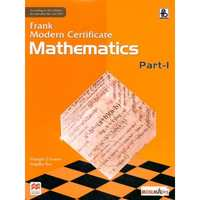## Chapter 24: Perimeter and Area

Exercise 24.1Exercise 24.2Exercise 24.3
Exercise 24.1

### Frank solutions for Class 9 Maths ICSE Chapter 24 Perimeter and Area Exercise 24.1

Exercise 24.1 | Q 1

Find the area of a triangle whose base is 3.8 cm and height is 2.8 cm.

Exercise 24.1 | Q 2

Find the area of a triangle whose sides are 27 cm, 45 cm and 36 cm.

Exercise 24.1 | Q 3

Find the area of an isosceles triangle ABC in which AB = AC = 6 cm, ∠A = 90°. Also, find the length of perpendicular from A to BC.

Exercise 24.1 | Q 4

Find the area of an equilateral triangle of side 20 cm.

Exercise 24.1 | Q 5

Find the perimeter of an equilateral triangle whose area is 16sqrt(3)"cm".

Exercise 24.1 | Q 6

Find the area of an equilateral triangle having perimeter of 18cm.

Exercise 24.1 | Q 7

Find the area of a triangle whose sides are in the ratio 5:12:13 and whose perimeter is 36cm.

Exercise 24.1 | Q 8

The perimeter of a triangle is 72cm and its sides are in the ratio 3:4:5. Find its area and the length of the altitude corresponding to the longest side.

Exercise 24.1 | Q 9

Find the area of a right angled triangle whose hypotenuse is 15cm and the base is 9cm.

Exercise 24.1 | Q 10

Find the area of the shaded region in the figure as shown, in which DPQS is an equilateral triangle and ∠PQR = 90°.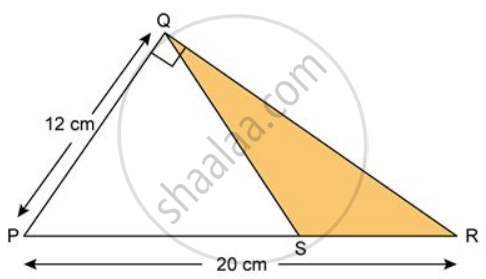Exercise 24.1 | Q 11

The area of an equilateral triangle is numerically equal to its perimeter. Find the length of its sides, correct two decimal places.

Exercise 24.1 | Q 12

In a right-angled triangle PQR right-angled at Q, QR = x cm, PQ = (x + 7) cm and area = 30 cm2. Find the sides of the triangle.

Exercise 24.1 | Q 13

In a right-angled triangle ABC, if ∠B = 90°, AB - BC = 2 cm; AC - BC = 4 and its perimeter is 24 cm, find the area of the triangle.

Exercise 24.1 | Q 13

In a right-angled triangle ABC, if ∠B = 90°, AB - BC = 2 cm; AC - BC = 4 and its perimeter is 24 cm, find the area of the triangle.

Exercise 24.1 | Q 14

Find the area of an isosceles triangle whose perimeter is 50cm and the base is 24cm.

Exercise 24.1 | Q 15

Find the area of an isosceles triangle whose perimeter is 72cm and the base is 20cm.

Exercise 24.1 | Q 16

The base of a triangle field and its height are in the ratio 2:5. If the cost of cultivating the field at Rs.42 per sq. m is Rs.7560, find its base and height.

Exercise 24.1 | Q 17

The cost of fencing a triangular field at the rate of Rs.15 per m is Rs.900. If the lengths of the triangle are in the ratio 3:4:5, find the area of the triangle and the cost of cultivating it at Rs.48 per kg sq.m.

Exercise 24.1 | Q 18

Find the area of a triangle with a base 12cm and a height equal to the width of a rectangle having area of 96cm2 and a length of 12cm.

Exercise 24.1 | Q 19

Find the base of an isosceles triangle whose area is 192cm2 and the length of one of the equal sides is 20cm.

Exercise 24.1 | Q 20

Each equal side of an isosceles triangle is 3cm less than the unequal side. The height of the perpendicular from the vertex to the unequal side is 3cm less than the equal side. If the area of the isosceles triangle is 108cm2, find the perimeter of the triangle.

Exercise 24.2

### Frank solutions for Class 9 Maths ICSE Chapter 24 Perimeter and Area Exercise 24.2

Exercise 24.2 | Q 1

The side of a square is of length 20 mm. Find its perimeter in cm.

Exercise 24.2 | Q 2

The area of a square is 36 cm2. How long are its sides?

Exercise 24.2 | Q 3

The sides of a rectangle are 5 cm and 3 cm respectively. Find its area in mm2.

Exercise 24.2 | Q 4

Find the area and perimeter of the given figure.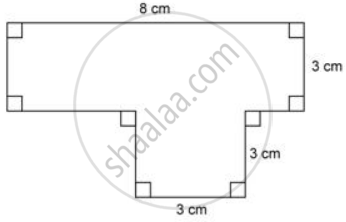Exercise 24.2 | Q 5

Find the shaded area in the given figure.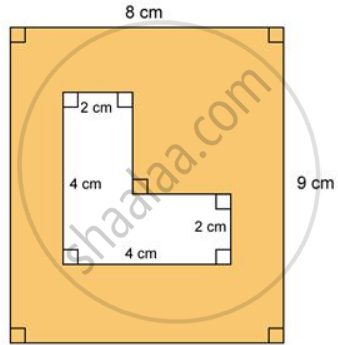Exercise 24.2 | Q 6.1

Find the area of each of the following figure: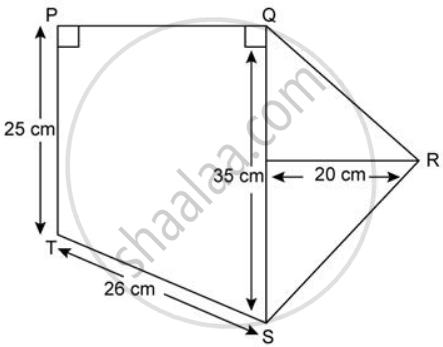Exercise 24.2 | Q 6.2

Find the area of each of the following figure: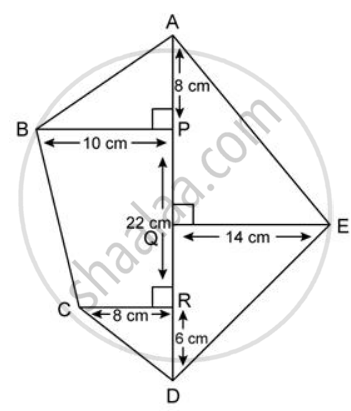Exercise 24.2 | Q 6.3

Find the area of each of the following figure: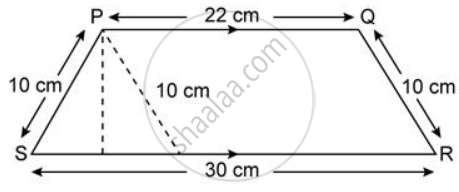Exercise 24.2 | Q 6.4

Find the area of each of the following figure: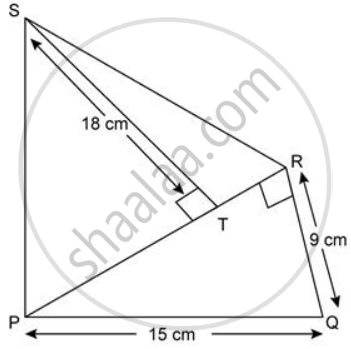Exercise 24.2 | Q 7

Find the area of a parallelogram whose base is 12cm and the height is 5cm.

Exercise 24.2 | Q 8

Find the height of a parallelogram whose area is 144cm2 and the base is 18cm.

Exercise 24.2 | Q 9

Find the area of quadrilateral, whose diagonals of lengths 18 cm and 13 cm intersect each other at right angle.

Exercise 24.2 | Q 10

In a rectangle ABCD, AB = 7 cm and AD = 25 cm. Find the height of a triangle whose base is AB and whose area is two times the area of the rectangle ABCD.

Exercise 24.2 | Q 11.1

Two adjacent sides of a parallelogram are 34 cm and 20 cm. If one of its diagonal is 42 cm, find: area of the parallelogram.

Exercise 24.2 | Q 11.2

Two adjacent sides of a parallelogram are 34 cm and 20 cm. If one of its diagonal is 42 cm, find: distance between its shorter sides

Exercise 24.2 | Q 12

One side of a parallelogram is 12cm and the altitude corresponding to i is 8cm. If the length of the altitude corresponding to its adjacent side is 16cm, find the length of the adjacent side.

Exercise 24.2 | Q 13

Two adjacent sides of a parallelogram are 20cm and 18cm. If the distance between the larger sides is 9cm, find the area of the parallelogram. Also, find the distance between the shorter sides.

Exercise 24.2 | Q 14

Find the perimeter and area of a rectangle whose length and breadth are 12cm and 9cm respectively.

Exercise 24.2 | Q 15

The area of a floor of a rectangular room is 360m2. If its length is 24cm, find its perimeter.

Exercise 24.2 | Q 16

The length of a rectangular field is thrice of its width. If the perimeter of this field is 1.6km, find its area in sq.m.

Exercise 24.2 | Q 17

A rectangular field 240m long has an area 36000m2. Find the cost of fencing the field at Rs.2.50per m.

Exercise 24.2 | Q 18

In a trapezium the parallel sides are 12cm and 8cm. If the distance between them is 6cm, find the area of the trapezium.

Exercise 24.2 | Q 19

A rectangular floor 45 in long and 12 m broad is to be paved exactly with square tiles, of side 60 cm. Find the total number of tiles required to pave it.
If a carpet is laid on the floor such as a space of 50 cm exists between its edges and the edges of the floor, find what fraction of the floor is uncovered.

Exercise 24.2 | Q 20

A rectangular hall of 40m by 24m is covered with carpets of size 6m x 4m. Find the number of carpets required to cover the hall.

Exercise 24.2 | Q 21

The area of a square garden is equal to the area of a rectangular plot of length 160m and width 40m. Calculate the cost of fencing the square garden at Rs.12per m.

Exercise 24.2 | Q 22

Find the area of a square whose diagonal is 12sqrt(12)"cm"

Exercise 24.2 | Q 23

Find the perimeter and area of a square whose diagonal is 5sqrt(2)"cm". Give your answer correct to two decimal places if sqrt(2) = 1.414.

Exercise 24.2 | Q 24

Find the perimeter of a rhombus whose diagonals are 24cm and 10cm.

Exercise 24.2 | Q 25

The perimeter of a square plot of land is 64m. The area of a nearby rectangular plot is 24m2 more than the area of the given square. If the length of the rectangle is 14m, find its breadth.

Exercise 24.2 | Q 26

The perimeter of a square is 128cm and that of another is 96cm. Find the perimeter and the diagonal of a square whose area is equal to the sum of the areas of these two squares.

Exercise 24.2 | Q 27

The area of a square plot of side 80m is equal to the area of a rectangular plot of length 160m. Calculate the width of the rectangular plot and the cost of fencing it Rs.7.50per m.

Exercise 24.2 | Q 28

The side of a square exceeds the side of another square by 4cm and the sum of the areas of the squares is 400cm2. Find the dimensions of the squares.

Exercise 24.2 | Q 29

Find the area of a rhombus, whose one side and one diagonal measure 20cm and 24cm respectively.

Exercise 24.2 | Q 30

The area of a rhombus is 234 cm2. If its one diagonal is 18 cm, find the lengths of its side and the other diagonal. Also, find perimeter of the rhombus.

Exercise 24.2 | Q 31

Find the area of a rhombus whose perimeter is 260cm and the length of one of its diagonal is 66cm.

Exercise 24.2 | Q 32

A rectangular field is 80m long and 50m wide. A 4m wide runs through the centre of the field parallel to the length and breadth of the field. Find the total area of the roads.

Exercise 24.2 | Q 33

A quadrilateral field of unequal has a longer diagonal with 140m. The perpendiculars from opposite vertives upon this diagonal are 20m and 14m. Find the area of the field.

Exercise 24.2 | Q 34

A lawn in the shape of a rectangle is to be developed in front of a Marriage Hall. The length and breadth of the lawn are 44m and 32m. A space of 2m is left on the two shoulder sides and one longer side for flower and in the remaining area grass is laid. Calculate the area of the flower space and the area on which grass is laid.

Exercise 24.2 | Q 35

The floor of a room is of size 6 m x 5 m. Find the cost of covering the floor of the room with 50 cm wide carpet at the rate of Rs.24.50 per metre. Also, find the cost of carpeting the same hall if the carpet, 60 cm, wide, is at the rate of Rs.26 per metre.

Exercise 24.2 | Q 36

A rectangular field is 240m long and 180m broad. In one corner a farm house is built on a square plot of side 40m. Find the area of the remaining portion and the cost of fencing the open sides Rs.25per m.

Exercise 24.2 | Q 37

Inside a square field of side 44m, a square flower bed is prepared leaving a graved path all round the flower bed. The total cost of laying the flower bed at Rs.25per  sq m. and gravelling the path at Rs.120per sq m. is Rs.80320. Find the width of the gravel path.

Exercise 24.2 | Q 38

The length and breadth of a rectangular field are in the ratio 8 : 5. A 2m wide path runs all around outside the field. The area of the path is 848m2. Find the length and breadth of the field.

Exercise 24.2 | Q 39

A footpath of uniform width runs all around the inside of a rectangular garden of 40 m x 30 m. If the path occupies 136 m2, find the width of the path.

Exercise 24.2 | Q 40

How many tiles, each of area 625 cm2, will be needed to pave a footpath which is 1 m wide and surrounds a grass plot of size 38 m x 14 m?

Exercise 24.2 | Q 41

PQRS is a square with each side 6cm. T is a point on QR such that the "area of ΔPQT"/"area of trapezium PTRS" = (1)/(3) Find the length of TR.

Exercise 24.2 | Q 42

The perimeter of a rectangular plot is 300m. It has an area of 5600m2. Taking the length of the plot as x m, calculate the breadth of the plot in terms of x, form an equation and solve it to find the dimensions of the plot.

Exercise 24.2 | Q 43

Find the area of a quadrilateral field whose sides are 12m, 9m, 18m and 21m respectively and the angle between the first two sides is a right angle. Take the value of sqrt(6) as 2.5.

Exercise 24.2 | Q 44

Find the diagonal of a quadrilateral whose area is 756cm2 and the perpendicular from the opposite vertices are 17cm and 19cm.

Exercise 24.2 | Q 45.1

A wire when bent in the form of a square encloses an area of 16 cm2. Find the area enclosed by it when the same wire is bent in the form of a rectangle whose sides are in the ratio of 1 : 3

Exercise 24.2 | Q 45.2

A wire when bent in the form of a square encloses an area of 16 cm2. Find the area enclosed by it when the same wire is bent in the form of an equilateral triangle

Exercise 24.2 | Q 46

A chessboard contains 64 equal square and the area of each square is 6.25cm2. A 2cm wide border is left inside of the board. Find the length of the side of the chessboard.

Exercise 24.2 | Q 47

The cross-section of a canal is a trapezium in shape. If the canal is 10m wide at the top, 6m wide at the bottom and the area of cross-section is 72 sq.m, determine its depth.

Exercise 24.3

### Frank solutions for Class 9 Maths ICSE Chapter 24 Perimeter and Area Exercise 24.3

Exercise 24.3 | Q 1.1

Find the area and perimeter of the circles with following: Radius = 2.8cm

Exercise 24.3 | Q 1.2

Find the area and perimeter of the circles with following: Radius = 10.5cm

Exercise 24.3 | Q 1.3

Find the area and perimeter of the circles with following: Diameter = 77cm

Exercise 24.3 | Q 1.4

Find the area and perimeter of the circles with following: Diameter = 35cm

Exercise 24.3 | Q 2.1

Find the area and perimeter of the following semicircles: Radius = 1.4cm

Exercise 24.3 | Q 2.2

Find the area and perimeter of the following semicircles: Diameter = 7cm

Exercise 24.3 | Q 2.3

Find the area and perimeter of the following semicircles: Diameter = 5.6cm

Exercise 24.3 | Q 3

Find the area of a circular field that has a circumference of 396m.

Exercise 24.3 | Q 4

Find the circumference of a circle whose area is 81πcm2.

Exercise 24.3 | Q 5

The diameter of a wheel is 1.4m. How many revolutions does make in moving a distance of 2.2km?

Exercise 24.3 | Q 6

The circumference of a circle exceeds its diameter by 450 cm. Find the area of the circle.

Exercise 24.3 | Q 7

The circumference of a circle is numerically equal to its area. Find the area and circumference of the circle.

Exercise 24.3 | Q 8

The sum of the circumference and diameter of a circle is 176 cm. Find the area of the circle.

Exercise 24.3 | Q 9

Find the radius and area of the circle which has circumference equal to the sum of circumferences of the two circles of radii 3 cm and 4 cm respectively.

Exercise 24.3 | Q 10

The diameter of two circles are 28 cm and 24 cm. Find the circumference of the circle having its area equal to sum of the areas of the two circles.

Exercise 24.3 | Q 11

The radii of two circles are in the ratio 5 : 8. If the difference between their areas is 156p cm2, find the area of the bigger circle.

Exercise 24.3 | Q 12

The diameters of three wheels are in the ratio 2 : 4 : 8. If the sum of the circumferences of these circles be 132 cm, find the difference between the areas of the largest and the smallest of these wheels.

Exercise 24.3 | Q 13

The wheel of a car makes 10 revolutions per second. If its diameter is 70cm, find the speed of the car in km per hr.

Exercise 24.3 | Q 14

The speed of a car is 66km per hour. If each wheel of the car is 140cm in diameter, find the number if revolution made by each wheel per minute.

Exercise 24.3 | Q 15

A cart wheel makes 9 revolutions per second. If the diameter of the wheel is 42cm, find its speed in km per hour. (Answer correct to the nearest km)

Exercise 24.3 | Q 16

A bucket is raised from a well by means of a rope wound round a wheel of diameter 35 cm. If the bucket ascends in 2 minutes with a uniform speed of 1.1 m per sec, calculate the number of complete revolutions the wheel makes in raising the bucket.

Exercise 24.3 | Q 17

The circumference o a garden roller is 280cm. How many revolutions does it make in moving 490m?

Exercise 24.3 | Q 18

The diameter of a cycle wheel is 4(5)/(11)"cm". How many revolutions will it make in moving 6.3km?

Exercise 24.3 | Q 19

The area of the circular ring enclosed between two concentric circles is 88 cm2. Find the radii of the two circles, if their difference is 1 cm.

Exercise 24.3 | Q 20

Find the area enclosed between two concentric circles, If their radii are 6cm and 13cm respectively.

Exercise 24.3 | Q 21

The area between the circumference of two concentric circles is 2464cm2. If the inner circle has a circumference of 132cm, calculate the radius of the outer circle.

Exercise 24.3 | Q 22

A wire when bent in the form of a square encloses an area of 484cm2. If the same wire is bent into the form of a circle, find the area of the circle.

Exercise 24.3 | Q 23

A wire bent in the form of an equilateral triangle has an area of 121sqrt(3)"cm"^2. If the same wire is bent into the form of a circle, find the area enclosed by the wire.

Exercise 24.3 | Q 24

Find the circumference of the circle whose area is 25times the area of the circle with radius 7cm.

Exercise 24.3 | Q 25

The circumference of a circle is equal to the perimeter of a square. The area of the square is 484 sq.m. Find the area of the circle.

Exercise 24.3 | Q 26

A wire in the form of a circle of radius 42cm. It is bent into a square. Determine the side of the square and compare the area of the regions enclosed in the two cases.

Exercise 24.3 | Q 27

A 7m wide road surrounds a circular garden whose area is 5544m2. Find the area of the road and the cost of tarring it at the rate of Rs.150 per m2.

Exercise 24.3 | Q 28

A 4.2m wide road surrounds a circular plot whose circumference is 176m. Find the cost of paving the road at Rs.75per m2.

Exercise 24.3 | Q 29

Two circles touch each other externally. The sum of their areas is 58πcm2 and the distance between their centres us 10cm. Find the radii of the two circles.

Exercise 24.3 | Q 30

The sum of diameters of two circles is 112cm and the sum of their areas is 5236cm2. Find the radii of the two circles.

Exercise 24.3 | Q 31

The sum of the radii of two circles is 10.5 cm and the difference of their circumferences is 13.2 cm. Find the radii of the two circles.

Exercise 24.3 | Q 32

A lawn is in the shape of a semicircle of diameter 42m. the lawn is surrounded by a flower bed of width 7m all round. Find the area of the flower bed in m2.

Exercise 24.3 | Q 33

A square is inscribed in a circle of radius 6 cm. Find the area of the square. Give your answer correct to two decimal places if sqrt(2) = 1.414.

Exercise 24.3 | Q 34

The cost of fencing a circular field at the rate of Rs.250 per metre is Rs.55000. The field is to be ploughing at the rate of Rs.15 per m2. Find the cost of ploughing the field.

Exercise 24.3 | Q 35

Find the area enclosed between two concentric circles of radii 6.3cm and 8.4cm. A third concentric circle is drawn outside the 8.4cm circle. So that the area enclosed between it and the 8.4cm circle is the same as that between the two inner circles. Find the radii of the third circle correct to two decimal places.

Exercise 24.3 | Q 36

Find the area of the biggest circle that can be cut from a rectangular piece 44cm by 28cm. also, find the area of the paper left after cutting out the circle.

## Chapter 24: Perimeter and Area

Exercise 24.1Exercise 24.2Exercise 24.3## Frank solutions for Class 9 Maths ICSE chapter 24 - Perimeter and Area

Frank solutions for Class 9 Maths ICSE chapter 24 (Perimeter and Area) include all questions with solution and detail explanation. This will clear students doubts about any question and improve application skills while preparing for board exams. The detailed, step-by-step solutions will help you understand the concepts better and clear your confusions, if any. Shaalaa.com has the CISCE Class 9 Maths ICSE solutions in a manner that help students grasp basic concepts better and faster.

Further, we at Shaalaa.com provide such solutions so that students can prepare for written exams. Frank textbook solutions can be a core help for self-study and acts as a perfect self-help guidance for students.

Concepts covered in Class 9 Maths ICSE chapter 24 Perimeter and Area are Some Special Types of Triangles - Equilateral and Isosceles Triangles, Area of Circle, Perimeter of Triangles, Area of a General Quadrilateral, Concept of Perimeter, Types of Quadrilaterals, Circumference of a Circle.

Using Frank Class 9 solutions Perimeter and Area exercise by students are an easy way to prepare for the exams, as they involve solutions arranged chapter-wise also page wise. The questions involved in Frank Solutions are important questions that can be asked in the final exam. Maximum students of CISCE Class 9 prefer Frank Textbook Solutions to score more in exam.

Get the free view of chapter 24 Perimeter and Area Class 9 extra questions for Class 9 Maths ICSE and can use Shaalaa.com to keep it handy for your exam preparation